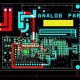# Best Interview Questions for Digital Electronics

In this tutorial we are going to learn about Best Interview Questions for Digital Electronics

Question1. What is Fan-in?

Answer – The number of input connected to a gate is called its fan-in.

Question2. What is Fan-out?

Answer – The number of gates to which the output of the given gate is connected as input.

Question3. What is Number System?

Answer – The number system, It is the system of naming or representing numbers. Generally have many types of number systems in maths like binary, decimal, etc.

Question4. What is Binary Encoding?

Answer – It is the representation of symbols in a source alphabet by strings of binary digits, i.e. a binary code. It is the alphabet consists of the set of alphanumeric characters.

Question5. What is K-Map?

Answer – K- Map means The Karnaugh map which provides a simple and straight-forward method of minimizing boo-lean expressions that represent combinational logic circuits.

Question6. What is Adder?

Answer – It is a digital circuit which performs addition of numbers. Mostly computers and other kinds of processors adders are used in the arithmetic logic units (ALU).

Question7. What is Subtractor?

Answer – It is an electronic logic circuit for calculating the difference between two binary numbers, which the minuend and the number to be subtracted.

Question8. What is Multiplexer?

Answer – This is also called data selector and it is a device which selects between several analog or digital input signals and forwards it to a single output line. A multiplexer of inputs has.

Question9. What is Decoder?

Answer –  It converts binary information from the n coded inputs to a maximum of 2n unique outputs and it is also called combination circuit.

Question10. What is Encoder?

Answer – It is encoder in digital electronics is a one-hot to binary converter. Generally, when there are 2ⁿ input lines.

Question11. What is Multiplexer?

Answer – It is work as a data selector and it is a device that selects between several analog or digital input signals and forwards it to a single output line. A multiplexer of inputs has.

Question12. What is De-Multiplexer?

Answer – This is a combinational logic circuit designed to switch one common input line to one of several separate output line.## About EEE

We have designing Experience for the last 40 years.